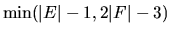Next: Extremal Combinatorics / Combinatoire Up: Discrete Geometry / Géométrie Previous: Asia Weiss - To

## Walter Whiteley - Constraining a spherical polyhedron with dihedral angles

 WALTER WHITELEY, Department of Mathematics and Statistics, York University, Toronto, Ontario  M3J 1P3, Canada Constraining a spherical polyhedron with dihedral angles

The theorems of Cauchy, Dehn and Alexandrov describe which distance constraints on edges and face diagonals of spherical polyhedra are independent and which are sufficient for (local) rigidity of convex polyhedra. We consider alternate constraints: the face-vertex incidences and the dihedral angles along edges. Specific results include:

(a)  the incidences alone are independent, and we then have at mostadditional independent dihedral angles;

(b)  dependencies of dihedral angles (or self-stresses of the corresponding spherical framework on the Gaussian sphere) are isomorphic to parallel drawings of the polyhedron with a fixed point (isogonal maps preserving all angles);

(c)  there are examples of additional dependencies which result in (infinitesimal) deformations of polyhedron preserving all incidences and dihedral angles--but not all face angles.

We conjecture that, for almost all proper realizations of any combinatorial type of spherical polyhedron with all dihedral angles fixed, there are only isogonal deformations.Next: Extremal Combinatorics / Combinatoire Up: Discrete Geometry / Géométrie Previous: Asia Weiss - To